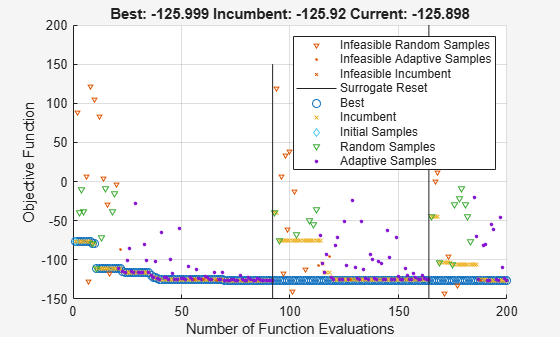# Surrogate Optimization with Nonlinear Constraint

This example shows how to include nonlinear inequality constraints in a surrogate optimization. The example solves an ODE with a nonlinear constraint. The example Optimize ODEs in Parallel shows how to solve the same problem using other solvers that accept nonlinear constraints.

For a video overview of this example, see Surrogate Optimization.

### Problem Description

The problem is to change the position and angle of a cannon to fire a projectile as far as possible beyond a wall. The cannon has a muzzle velocity of 300 m/s. The wall is 20 m high. If the cannon is too close to the wall, it fires at too steep an angle, and the projectile does not travel far enough. If the cannon is too far from the wall, the projectile does not travel far enough.

Nonlinear air resistance slows the projectile. The resisting force is proportional to the square of velocity, with the proportionality constant 0.02. Gravity acts on the projectile, accelerating it downward with constant 9.81 m/s^2. Therefore, the equations of motion for the trajectory x(t) are

`$\frac{{d}^{2}x\left(t\right)}{d{t}^{2}}=-0.02‖v\left(t\right)‖v\left(t\right)-\left(0,9.81\right),$`

where $v\left(t\right)=dx\left(t\right)/dt$.

The initial position `x0` and initial velocity `xp0` are 2-D vectors. However, the initial height `x0(2)` is 0, so the initial position is given by the scalar `x0(1)`. The initial velocity has magnitude 300 (the muzzle velocity) and, therefore, depends only on the initial angle, which is a scalar. For an initial angle `th`, the initial velocity is `xp0 = 300*(cos(th),sin(th))`. Therefore, the optimization problem depends only on two scalars, making it a 2-D problem. Use the horizontal distance and initial angle as the decision variables.

### Formulate ODE Model

ODE solvers require you to formulate your model as a first-order system. Augment the trajectory vector $\left({x}_{1}\left(t\right),{x}_{2}\left(t\right)\right)$ with its time derivative $\left({x}_{1}\prime \left(t\right),{x}_{2}\prime \left(t\right)\right)$ to form a 4-D trajectory vector. In terms of this augmented vector, the differential equation becomes

`$\frac{\mathit{d}}{\mathit{dt}}\mathit{x}\left(\mathit{t}\right)=\left[\begin{array}{c}{\mathit{x}}_{3}\left(\mathit{t}\right)\\ {\mathit{x}}_{4}\left(\mathit{t}\right)\\ -0.02‖\left({\mathit{x}}_{3}\left(\mathit{t}\right),{\mathit{x}}_{4}\left(\mathit{t}\right)\right)‖{\mathit{x}}_{3}\left(\mathit{t}\right)\\ -0.02‖\left({\mathit{x}}_{3}\left(\mathit{t}\right),{\mathit{x}}_{4}\left(\mathit{t}\right)\right)‖{\mathit{x}}_{4}\left(\mathit{t}\right)-9.81\end{array}\right].$`

The `cannonshot` file implements this differential equation.

`type cannonshot`
```function f = cannonshot(~,x) f = [x(3);x(4);x(3);x(4)]; % initial, gets f(1) and f(2) correct nrm = norm(x(3:4)) * .02; % norm of the velocity times constant f(3) = -x(3)*nrm; % horizontal acceleration f(4) = -x(4)*nrm - 9.81; % vertical acceleration ```

Visualize the solution of this ODE starting 30 m from the wall with an initial angle of `pi/3`. The `plotcannonsolution` function uses `ode45` to solve the differential equation.

`type plotcannonsolution`
```function dist = plotcannonsolution(x) % Change initial 2-D point x to 4-D x0 x0 = [x(1);0;300*cos(x(2));300*sin(x(2))]; sol = ode45(@cannonshot,[0,15],x0); % Find the time when the projectile lands zerofnd = fzero(@(r)deval(sol,r,2),[sol.x(2),sol.x(end)]); t = linspace(0,zerofnd); % equal times for plot xs = deval(sol,t,1); % interpolated x values ys = deval(sol,t,2); % interpolated y values plot(xs,ys) hold on plot([0,0],[0,20],'k') % Draw the wall xlabel('Horizontal distance') ylabel('Trajectory height') ylim([0 100]) legend('Trajectory','Wall','Location','NW') dist = xs(end); title(sprintf('Distance %f',dist)) hold off ```

`plotcannonsolution` uses `fzero` to find the time when the projectile lands, meaning its height is 0. The projectile lands before time 15 s, so `plotcannonsolution` uses 15 as the amount of time for the ODE solution.

```x0 = [-30;pi/3]; dist = plotcannonsolution(x0);```### Prepare Optimization

To optimize the initial position and angle, write a function similar to the previous plotting routine. Calculate the trajectory starting from an arbitrary horizontal position and initial angle.

Include sensible bound constraints. The horizontal position cannot be greater than 0. Set an upper bound of –1. Similarly, the horizontal position cannot be below –200, so set a lower bound of –200. The initial angle must be positive, so set its lower bound to 0.05. The initial angle should not exceed `pi`/2; set its upper bound to `pi`/2 – 0.05.

```lb = [-200;0.05]; ub = [-1;pi/2-.05];```

Write an objective function that returns the negative of the resulting distance from the wall, given an initial position and angle. If the trajectory crosses the wall at a height less than 20, the trajectory is infeasible; this constraint is a nonlinear constraint. The `cannonobjcon` function implements the objective function calculation. To implement the nonlinear constraint, the function calls `fzero` to find the time when the x-value of the projectile is zero. The function accounts for the possibility of failure in the `fzero` function by checking whether, after time 15, the x-value of the projectile is greater than zero. If not, then the function skips the step of finding the time when the projectile passes the wall.

`type cannonobjcon`
```function f = cannonobjcon(x) % Change initial 2-D point x to 4-D x0 x0 = [x(1);0;300*cos(x(2));300*sin(x(2))]; % Solve for trajectory sol = ode45(@cannonshot,[0,15],x0); % Find time t when trajectory height = 0 zerofnd = fzero(@(r)deval(sol,r,2),[1e-2,15]); % Find the horizontal position at that time dist = deval(sol,zerofnd,1); % What is the height when the projectile crosses the wall at x = 0? if deval(sol,15,1) > 0 wallfnd = fzero(@(r)deval(sol,r,1),[0,15]); height = deval(sol,wallfnd,2); else height = deval(sol,15,2); end f.Ineq = 20 - height; % height must be above 20 % Take negative of distance for maximization f.Fval = -dist; end ```

You already calculated one feasible initial trajectory. Use that value as an initial point.

```fx0 = cannonobjcon(x0); fx0.X = x0;```

### Solve Optimization Using `surrogateopt`

Set `surrogateopt` options to use the initial point. For reproducibility, set the random number generator to `default`. Use the `'surrogateoptplot'` plot function. Run the optimization. To understand the `'surrogateoptplot'` plot, see Interpret surrogateoptplot.

```opts = optimoptions('surrogateopt','InitialPoints',x0,'PlotFcn','surrogateoptplot'); rng default [xsolution,distance,exitflag,output] = surrogateopt(@cannonobjcon,lb,ub,opts)``````surrogateopt stopped because it exceeded the function evaluation limit set by 'options.MaxFunctionEvaluations'. ```
```xsolution = 1×2 -28.4012 0.6161 ```
```distance = -125.9987 ```
```exitflag = 0 ```
```output = struct with fields: elapsedtime: 53.4358 funccount: 200 constrviolation: 8.0630e-04 ineq: 8.0630e-04 rngstate: [1x1 struct] message: 'surrogateopt stopped because it exceeded the function evaluation limit set by ...' ```

Plot the final trajectory.

```figure dist = plotcannonsolution(xsolution);```The `patternsearch` solution in Optimize ODEs in Parallel shows a final distance of `125.9880`, which is almost the same as this `surrogateopt` solution.